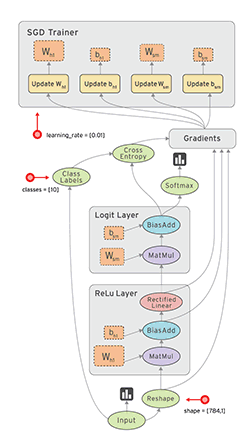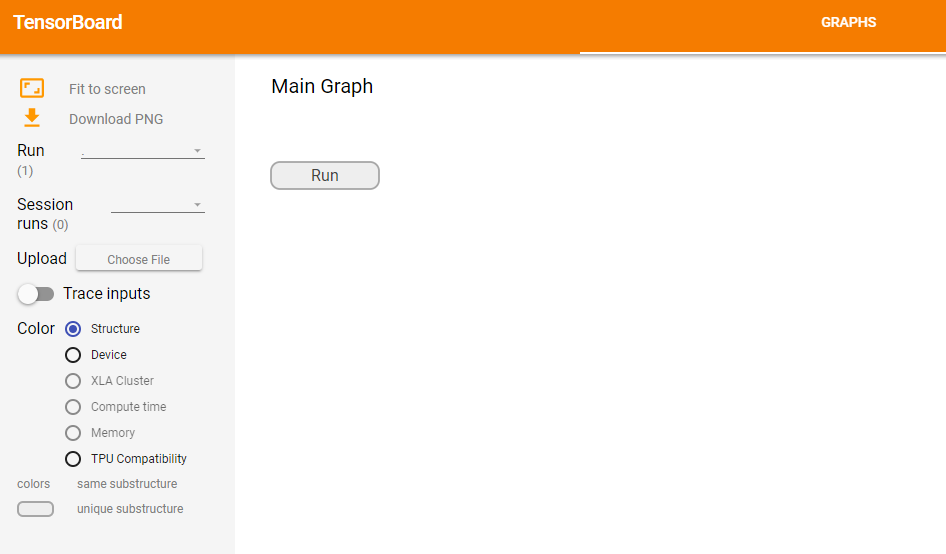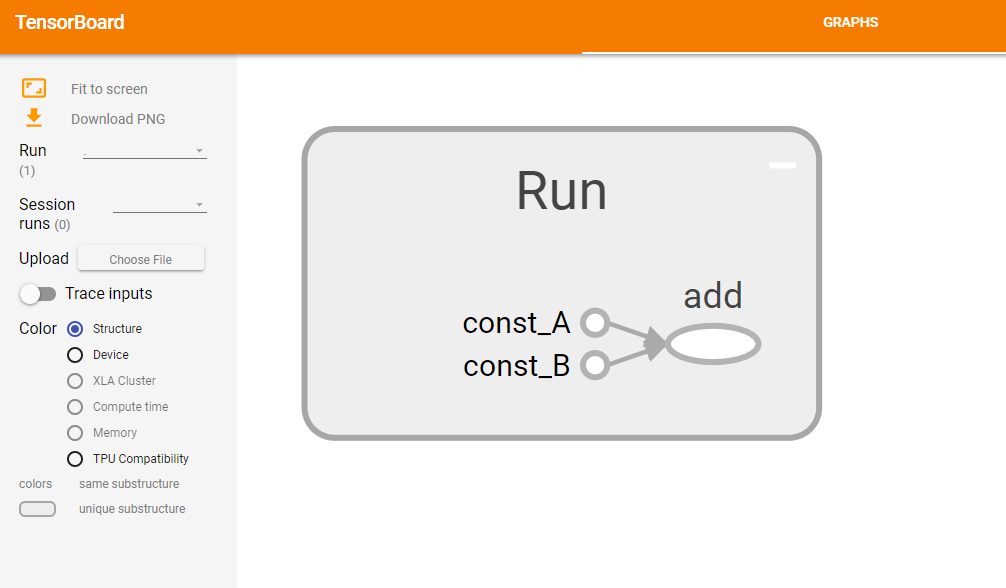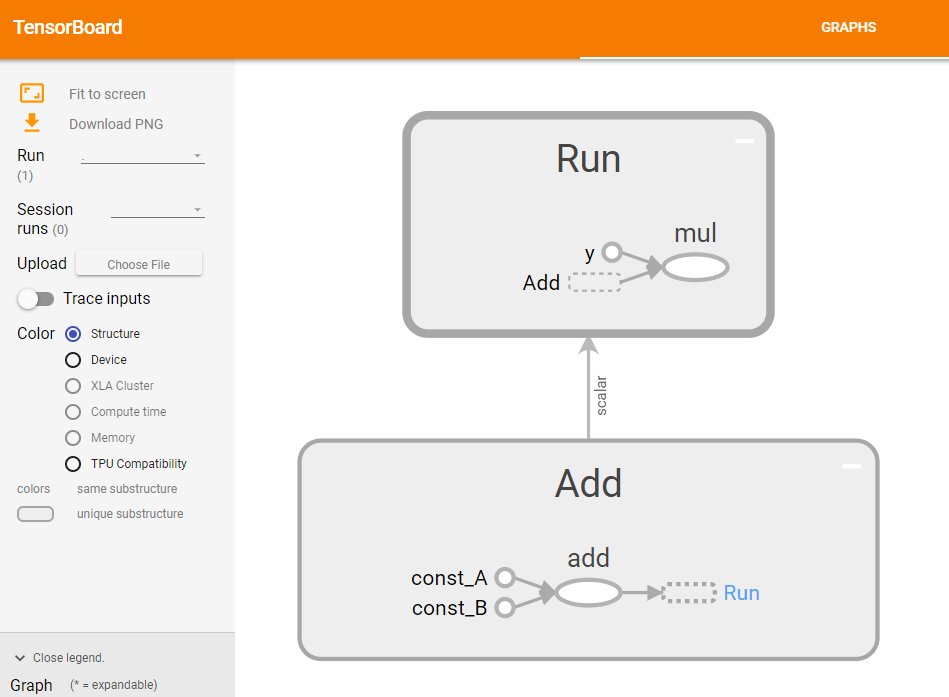## 目次

【Python】TensorFlow學習筆記(一)：TensorBoard 的浪漫
【Python】TensorFlow學習筆記(二)：初探 TFRecord
【Python】TensorFlow學習筆記(三)：再探 TFRecord
【Python】TensorFlow學習筆記(四)：用 TFRecord 餵食 Softmax Model
【Python】TensorFlow學習筆記(五)：存檔 & 讀檔
【Python】TensorFlow學習筆記(六)：卷積的那些小事​
【Python】TensorFlow學習筆記(完)：卷積深深深幾許

## 什麼是 TensorFlow?

TensorFlow 是一個採用資料流程圖(data flow graphs)，用於數值計算的開源軟體庫。## TensorFlow

Python版本：python-3.5
TensorFlow版本：tensorflow-1.5.0

TensorFlow 的安裝方法很簡單，請直接在指令列輸入：

# pip install --ignore-installed --upgrade tensorflow            或是
# pip install --ignore-installed --upgrade tensorflow-gpu

https://www.tensorflow.org/install/

【Python】在 Win10 系統下建立影像處理及深度學習環境

import tensorflow as tf

# constant可以視為tf專用的變數型態
# 其他包括Variable，placeholder
A = tf.constant('Hello World!')

# 使用 with 可以讓Session自動關閉
with tf.Session() as sess:

# 在 tensorflow內要使用run，才會讓計算圖開始執行
B = sess.run(A)

print(B)import os
os.environ['TF_CPP_MIN_LOG_LEVEL'] = '2'

## tf.constant

# -*- coding: utf-8 -*-

import tensorflow as tf

# 宣告常數
A = tf.constant(10, dtype=tf.int64)

with tf.Session() as sess:
print( A )
# Tensor("Const_42:0", shape=(), dtype=int64)

print( sess.run(A) )
# 10

# 使用 sess.run() 才能取得 A 的值

## tf.Variable

# -*- coding: utf-8 -*-

import tensorflow as tf

# 宣告變數
B = tf.Variable(0, dtype=tf.int64)
with tf.Session() as sess:
# 變數需要初始化
sess.run( tf.global_variables_initializer() )

# 使用 assign 更改變數值
for i in range(5):
print( sess.run(B.assign(i)) )

'''
執行結果：
0
1
2
3
4
'''

for i in range(5):
B.assign(i)
print( sess.run(B) )

## tf.placeholder

# -*- coding: utf-8 -*-

import tensorflow as tf

# 宣告占位符
C = tf.placeholder(dtype=tf.int64)

with tf.Session() as sess
for i in range(5):
result = sess.run(C, feed_dict={C:i})
print(result)

# -*- coding: utf-8 -*-

import tensorflow as tf

# 宣告占位符
C = tf.placeholder(dtype=tf.int64)
D = tf.placeholder(dtype=tf.int64)
E = tf.placeholder(dtype=tf.int64)

F = D + E

with tf.Session() as sess:
# 可以一次填充所有的占位符
result = sess.run(F, feed_dict={C:10, D:20, E:30})
print(result) # 50

# 或者只填充計算所需要的
result = sess.run(F, feed_dict={D:20, E:30})
print(result) # 50

# 這段程式會使系統報錯！
# 計算所需的占位符不能為空，每次執行 sess.run() 都要填充，
# 占位符不是變數，不會留存上次填充的資料。
result = sess.run(F, feed_dict={E:30})
print(result)

## TensorBoard

...扯遠了。

#### 2. 接著使用 tf.summary.FileWriter 函數輸出到目標資料夾。

import tensorflow as tf

# 宣告常數A&B，後面的name參數，是要繪製tensorboard時所使用的名稱。
# 若沒有指定，或是重複名稱，則tensorboard會自動修改。
A = tf.constant(50, name='const_A')
B = tf.constant(100, name='const_B')

with tf.Session() as sess:
# 就是這邊！
# 使用 "with tf.name_scope('Run'):" 這句話可以畫出Run這個步驟。
with tf.name_scope('Run'):
B = sess.run(A+B)
print(B)

# 畫好步驟之後，要使用"tf.summary.FileWriter"把檔案寫到目標資料夾，
# 第二個參數表示要把整個圖層放到graph參數內，這樣才能用tensorboard畫出來。
train_writer = tf.summary.FileWriter('/home/shayne/tfboard_Test', sess.graph)
train_writer.close()

events.out.tfevents.xxxxxxxxxx(一連串的數字).(主機名稱)

$tensorboard --logdir=/home/shayne/tfboard_Test 這個指令的格式是：$ tensorboard --logdir=(your path)

# service firewalld restartimport tensorflow as tf

A = tf.constant(50, name='const_A')
B = tf.constant(100, name='const_B')

C = A+B

with tf.Session() as sess:
with tf.name_scope('Run'):
D = sess.run(C*3)
print(D)

train_writer = tf.summary.FileWriter('/home/shayne/tfboard_Test', sess.graph)
train_writer.close()TensorBoard 除了可以繪製計算圖之外，還能夠記錄在訓練過程中的參數變化及展示訓練資料庫的影像。

https://www.tensorflow.org/get_started/summaries_and_tensorboard

TensorFlow 真的是一個又難搞又功能強大的東西，讓人又愛又恨的！

【Python】TensorFlow學習筆記(二)：初探 TFRecord